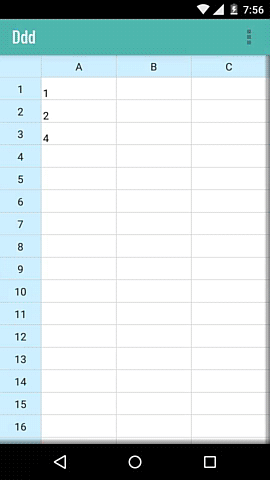Formulas use the same syntax as used in excel. The easiest way to add formulas is with the formula helper. To access it, touch the formatting button to open up the formatting menu.

• Press on the cell you want to add the formula on.
• Use the formatting icon on top to bring up the formatting menu at bottom.
• Select the formula and then proceed to select the input cells.If you want to type formulas yourself, here's a list you can use. All formulas start with an "=" sign.

• =SUM(A1,B1) - Sums two cells.
• =SUM(A1:A5) - Sums a range of cells.
• =MINUS(A1,B1) Subtracts the second cell from first.
• =MULTIPLY(A1,B1) - Multiplies two cells.
• =DIVIDE(A1,B1) - Divides first cell by second.
• =COUNT(A1:A5) - Count of non-empty cells between the two cells.
• =AVERAGE(A1:A5) - Average of values of a range of cells.
• =MAX(A1:A5) - Maximum of values of a range of cells.
• =MIN(A1:A5) - Minimum of values of a range of cells.
• =PERCENTAGE(A1:A5,50) - Average percentage of values in cells A1 to A5 considering 50 as max for each cell.